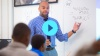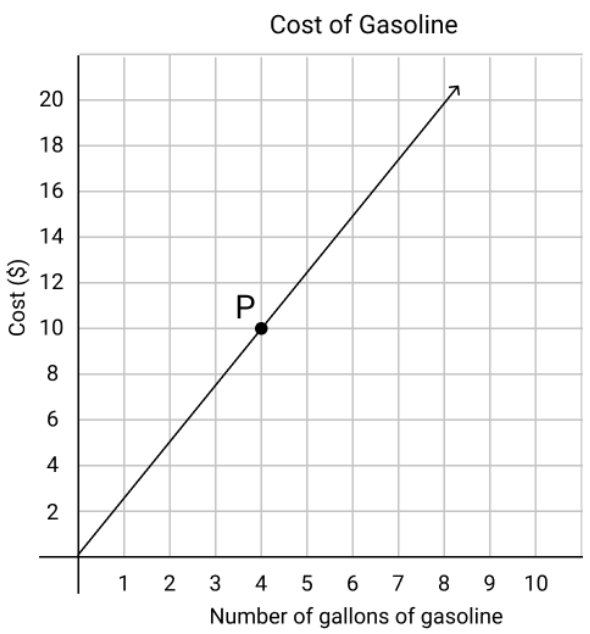# Proportional Relationships

Students deepen their understanding of ratios to investigate proportional relationships, in order to solve multi-step, real-world ratio problems using new strategies that rely on proportional reasoning.

Math

Unit 1

## Unit Summary

In Unit 1, 7th grade students deepen their understanding of ratios to investigate and analyze proportional relationships. They begin the unit by looking at how proportional relationships are represented in tables, equations, and graphs. As they analyze each representation, students continue to internalize what proportionality means, and how concepts like the constant of proportionality are visible in different ways. Students then spend time comparing examples of proportional and non-proportional associations, and studying how all the representations are connected to one another. Finally, in this unit, students will solve multi-step, real-world ratio and rate problems using efficient strategies and representations that rely on proportional reasoning (MP.4). These new strategies and representations, such as setting up and solving a proportion, are added to students’ growing list of approaches to solve problems. Throughout the unit, students will engage with MP.2 and MP.6. Translating between equations, graphs, tables, and written explanations requires students to reason both abstractly and quantitatively, and to pay precise attention to units, calculations, and forms of communication throughout their work.

In 6th grade, students were introduced to the concept of ratios and rates. They learned several strategies to represent ratios and to solve problems, including using concrete drawings, double number lines, tables, tape diagrams, and graphs. They defined and found unit rates and applied this to measurement conversion problems. 7th grade students will draw on these conceptual understandings to fully understand proportional relationships.

Beyond this unit, in Unit 5, students will re-engage with proportional reasoning, solving percent problems and investigating how proportional reasoning applies to scale drawings. In eighth grade, students connect unit rate to slope, and they compare proportional relationships across different representations. They expand their understanding of non-proportional relationships to study linear functions in the form of $$y=mx+b$$, and compare these to non-linear functions, such as $$y=6x^2$$.

Pacing: 22 instructional days (18 lessons, 3 flex days, 1 assessment day)

Fishtank Plus for Math

Unlock features to optimize your prep time, plan engaging lessons, and monitor student progress.## Assessment

The following assessments accompany Unit 1.

### Pre-Unit

Have students complete the Pre-Unit Assessment and Pre-Unit Student Self-Assessment before starting the unit. Use the Pre-Unit Assessment Analysis Guide to identify gaps in foundational understanding and map out a plan for learning acceleration throughout the unit.

### Mid-Unit

Have students complete the Mid-Unit Assessment after lesson 9.

### Post-Unit

Use the resources below to assess student understanding of the unit content and action plan for future units.

Expanded Assessment Package

Use student data to drive your planning with an expanded suite of unit assessments to help gauge students’ facility with foundational skills and concepts, as well as their progress with unit content.

## Unit Prep

### Intellectual Prep

Unit Launch

Prepare to teach this unit by immersing yourself in the standards, big ideas, and connections to prior and future content. Unit Launches include a series of short videos, targeted readings, and opportunities for action planning.#### Internalization of Standards via the Post-Unit Assessment

• Take the Post-Unit Assessment. Annotate for:
• Standards that each question aligns to
• Strategies and representations used in daily lessons
• Relationship to Essential Understandings of unit
• Lesson(s) that Assessment points to

#### Internalization of Trajectory of Unit

• Read and annotate the Unit Summary.
• Notice the progression of concepts through the unit using the Lesson Map.
• Essential Understandings
• Connection to Post-Unit Assessment questions
• Identify key opportunities to engage students in academic discourse. Read through our Teacher Tool on Academic Discourse and refer back to it throughout the unit.

#### Unit-Specific Intellectual Prep

Model Example
Setting up and solving a proportion

A group of 4 students buy movie tickets for $24. At this rate, how much would 20 students pay for the movie? $$\frac{4\space \mathrm{students}}{24} = \frac{20\space \mathrm{students}}{x}$$ $$4x=24(20)$$ $$x=120$$ Table of equivalent ratios The table below shows some weights of rice, in pounds, and their corresponding costs, in dollars.  Rice (lbs.) Cost ($) 2 11 10 55 13 ? ? 88
Equation The equation $$y=8.75x$$ represents the cost in dollars, $$y$$, to purchase $$x$$ pounds of turkey meat at a deli.
Graph

The graph below shows the relationship between the cost of gas and the number of gallons of gas purchased at a gas station.### Essential Understandings

• A proportional relationship between two quantities is a collection of equivalent ratios, related to each other by a constant of proportionality.
• Proportional relationships can be represented in different, related ways, including a table, equation, graph, and written description. Knowing one representation provides the information needed to represent the relationship in a different way.
• A unit rate, associated with a ratio $$a:b$$, is $$a/b$$ or $$b/a$$ units of one quantity per 1 unit of another quantity. Unit rates are represented in equations of the form $${y=kx}$$ and in graphs of proportional relationships as the ordered pair $$(1, r)$$.
• There are many applications that can be solved using proportional reasoning, including problems with price increases and decreases, commissions, fees, unit prices, and constant speed.

### Vocabulary

commission

constant of proportionality

dependent variable

equivalent ratio

independent variable

part to whole ratio

part to part ratio

proportion

proportional relationship

ratio

rate

unit rate

To see all the vocabulary for Unit 1, view our 7th Grade Vocabulary Glossary.

### Materials

• Calculators (1 per student)
• Graph Paper (1 per student)
• Ruler (2-3 sheets per student)

To see all the materials needed for this course, view our 7th Grade Course Material Overview.

## Lesson Map

Topic A: Representing Proportional Relationships in Tables, Equations, and Graphs

Topic B: Non-Proportional Relationships

Topic C: Connecting Everything Together

Topic D: Solving Ratio & Rate Problems with Fractions

## Common Core Standards

Key

Major Cluster

Supporting Cluster

### Core Standards

#### Ratios and Proportional Relationships

• 7.RP.A.1 — Compute unit rates associated with ratios of fractions, including ratios of lengths, areas and other quantities measured in like or different units. For example, if a person walks 1/2 mile in each 1/4 hour, compute the unit rate as the complex fraction 1/2/1/4 miles per hour, equivalently 2 miles per hour.
• 7.RP.A.2 — Recognize and represent proportional relationships between quantities.
• 7.RP.A.2.A — Decide whether two quantities are in a proportional relationship, e.g., by testing for equivalent ratios in a table or graphing on a coordinate plane and observing whether the graph is a straight line through the origin.
• 7.RP.A.2.B — Identify the constant of proportionality (unit rate) in tables, graphs, equations, diagrams, and verbal descriptions of proportional relationships.
• 7.RP.A.2.C — Represent proportional relationships by equations. For example, if total cost t is proportional to the number n of items purchased at a constant price p, the relationship between the total cost and the number of items can be expressed as t = pn.
• 7.RP.A.2.D — Explain what a point (x, y) on the graph of a proportional relationship means in terms of the situation, with special attention to the points (0, 0) and (1, r) where r is the unit rate.
• 7.RP.A.3 — Use proportional relationships to solve multistep ratio and percent problems. Examples: simple interest, tax, markups and markdowns, gratuities and commissions, fees, percent increase and decrease, percent error.

• 6.EE.B.7
• 6.EE.C.9

• 5.NF.B.6

• 6.RP.A.1
• 6.RP.A.2
• 6.RP.A.3
• 6.RP.A.3.A
• 6.RP.A.3.B

• 6.NS.A.1

• 8.EE.B.5
• 8.EE.B.6

• 8.F.A.1
• 8.F.A.2
• 8.F.A.3
• 8.F.B.4
• 8.F.B.5

### Standards for Mathematical Practice

• CCSS.MATH.PRACTICE.MP1 — Make sense of problems and persevere in solving them.

• CCSS.MATH.PRACTICE.MP2 — Reason abstractly and quantitatively.

• CCSS.MATH.PRACTICE.MP3 — Construct viable arguments and critique the reasoning of others.

• CCSS.MATH.PRACTICE.MP4 — Model with mathematics.

• CCSS.MATH.PRACTICE.MP5 — Use appropriate tools strategically.

• CCSS.MATH.PRACTICE.MP6 — Attend to precision.

• CCSS.MATH.PRACTICE.MP7 — Look for and make use of structure.

• CCSS.MATH.PRACTICE.MP8 — Look for and express regularity in repeated reasoning.

Unit 2

Operations with Rational Numbers

## Request a Demo

See all of the features of Fishtank in action and begin the conversation about adoption.

Yes

No

### Any other information you would like to provide about your school?